### REGENCY INSIGHTS BLOG

Practical advice on commercial lighting from LED retrofts to lighting design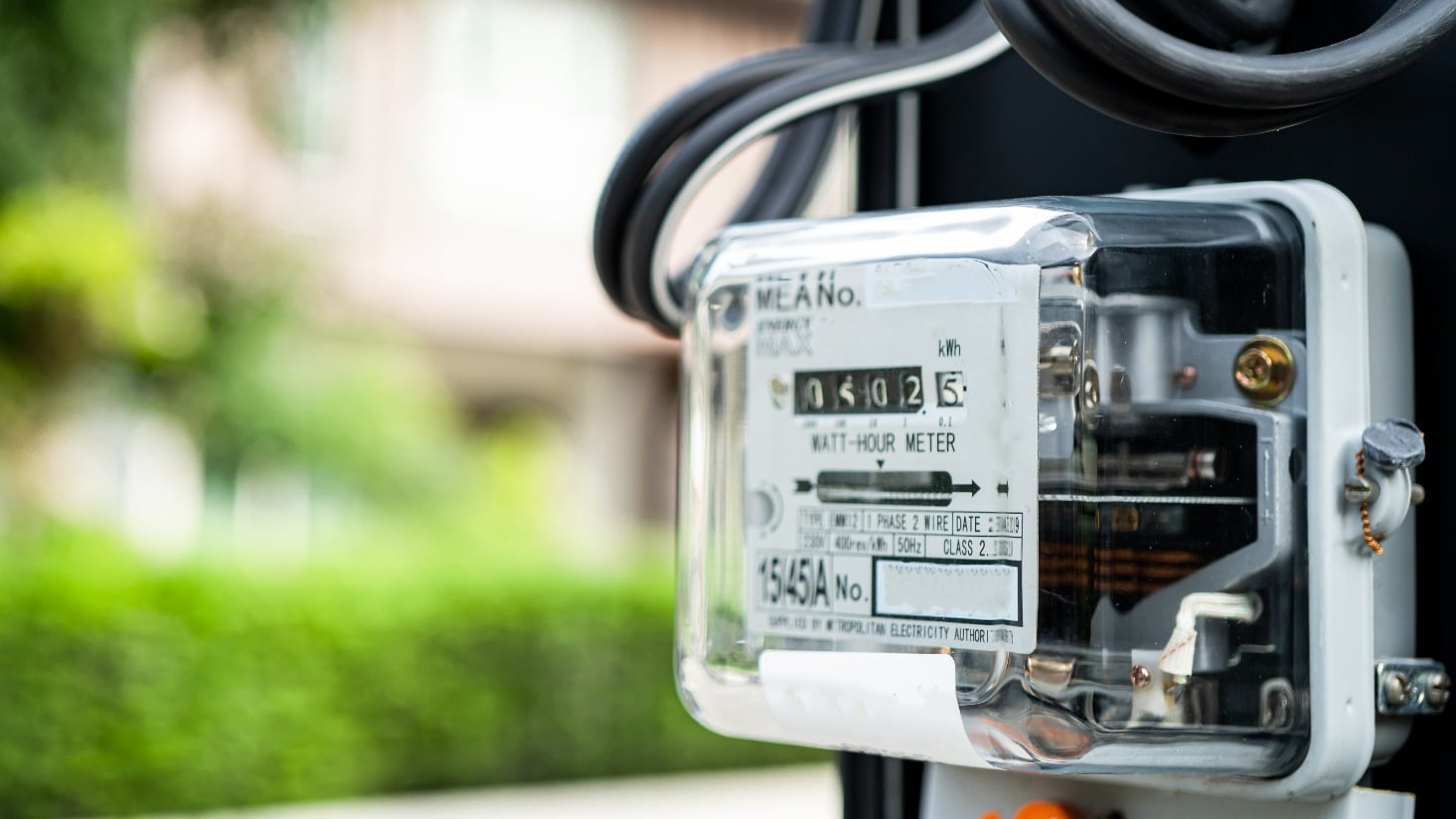# kW vs. kWh: How much energy is my lighting using? [Calculator]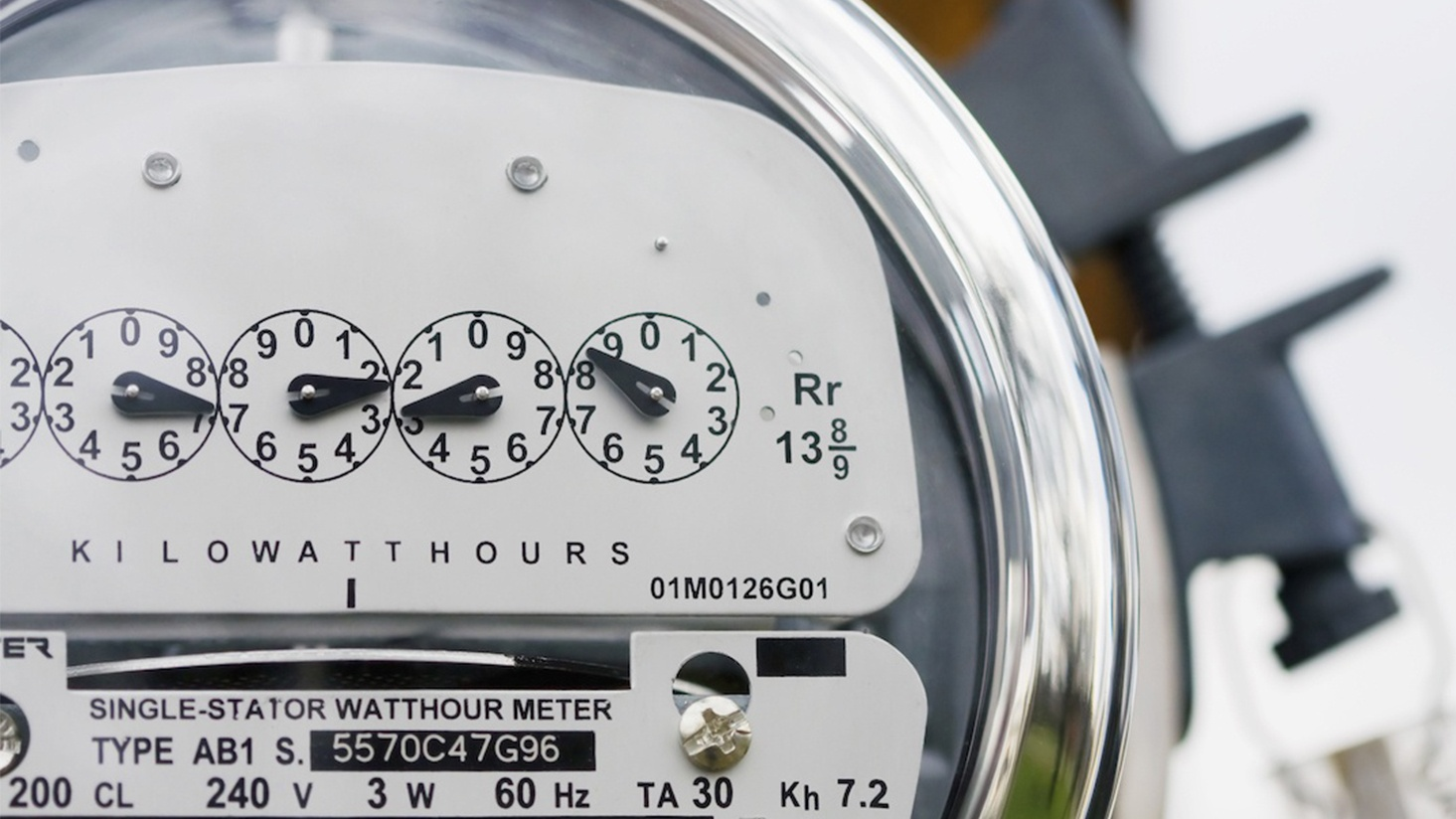How much energy does a light bulb use, and how does lighting affect your electricity bill? How much will a lighting retrofit save you? How do you begin to estimate the value of a potential lighting rebate?

How does lighting affect your electricity bill? How much will a lighting retrofit save you? How do you begin to estimate the value of a potential lighting rebate?

There’s one common thread that runs through each of these questions: the difference between watts (W), kilowatts (kW), and kilowatt-hours (kWh). Calculating watts to kWh could help you understand the answers to the questions above.

The energy industry is almost as bad as the lighting industry at using abbreviations and jargon, so we’ll try to break each of these down with some practical examples.

In this article, I’m going to use the analogy of equating electricity to water. This is a common analogy that we can’t claim as our own, but hopefully our specific examples will help explain how much energy lighting really uses.## What is a watt (W)?

Before LEDs hit the market, you probably made a lot of lighting decisions based on wattage. You unscrewed a burned out light bulb and saw “60W” at the top. All you had on hand was a light bulb labeled “25W,” so you screwed it in, and it was way too dim. You went to the store and picked out a “60W” bulb. Your lights were bright again. Crisis averted. But that's not the case anymore with more efficient lighting (more on that below).

So what is a watt, anyway? Technically speaking, it's a unit of electrical power equal to 1 joule per second. Light bulbs are rated in watts to indicate how much energy they consume.

Does the wattage of a light bulb have anything to do with brightness? Well, sort of.

For so long, many of us have associated watts with the amount of light a bulb gives off. In general, that works well with traditional incandescent light bulbs. A 60W incandescent bulb typically gives off around 650-800 lumens. A 25W incandescent bulb typically produces around 150 lumens — much less light.

But now, with federal regulations requiring more efficient lighting, there is a focus on “60W equivalent” bulbs that use far less energy and gives off roughly the same amount of light. Here’s a breakdown comparing wattages:

 Incandescent Halogen CFL LED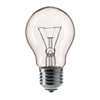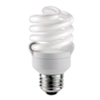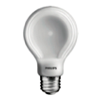Wattage 60W 42W 13-16W 5-9W Lumens per watt (LPW) 13 18.5 60 75-100+

(The photos above are representative of the technology, not specific product specifications.)

So, as you are comparing light bulbs, remember that wattage is a measure of how much energy the light bulb will use as it produces light, and the lumens will give you a measure of how much light it will produce.

Note: Starting in August 2023, many incandescent and halogen light bulbs will no longer be sold. CFLs are also restricted in some states. To stay up to date, click here.

## What is a kilowatt (kW)?

Just like watts, kilowatts is a measure of how much energy something will consume. Going from watts (W) to kilowatts (kW) is a pretty straightforward calculation: 1kW is equal to 1,000W. To convert W to kW, divide the total wattage by 1,000.

Here’s an example: if you turned on ten 100W light bulbs, that would equal 1kW of energy usage.

10 bulbs x 100W = 1,000W

1,000W / 1,000 = 1kW

It’s also worth noting that kW can be synonymous with “demand” if you’re talking to a utility company. Imagine that you turn on those ten light bulbs and a 3,000-watt clothes dryer at the same time. The utility company needs to be able to supply enough electricity to meet that 4kW of demand at the moment you turn everything on.

Your energy usage, though, depends on how long you keep everything on, which leads us to…

## What is a kilowatt-hour (kWh)?

What’s the difference between kW and kWh? The kWh measurement is a way to quantify how much energy is used over a period of time. This can be calculated by multiplying the kW of energy consumption by the total number of hours the lighting has been operated.

Let’s go back to the example of having ten 100W light bulbs. How much energy would you use over the course of a month if you turn them on for 10 hours a day?

That’s where kWh comes into play. Here’s the breakdown:

10 bulbs X 100W = 1,000W or 1kW of lighting

10 hours of daily use X 30 days in the month = 300 hours of use

1kW X 300 hours of use = 300kWh of energy consumption

So why is kWh so important if you can compare lighting products based on wattage and light output? In the end, a significant portion of your electricity bill is based on your energy consumption in kilowatt-hours. On other words, the amount you pay is typically tied to how often you have the light on. If you want to calculate the dollar savings you’ll get from a retrofit to more efficient lighting, kWh will come into play.

## kW vs kWh: A practical example

Okay, enough theory.

How about a practical example to explain the difference between kW and kWh? (Here’s where the water analogy comes in.)

Let’s imagine that we have two garden hoses, one with a 3/8-inch diameter and one with a 5/8-inch diameter.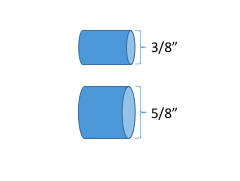The 5/8-inch hose has a larger capacity than the 3/8-inch hose, so it can carry more water at any given moment. This is the idea of kW — the capacity to use electricity.

Now, let’s imagine that we want to fill up a two-gallon watering can. The amount of water that we use to fill up the can is two gallons. This is the idea of kWh — the total amount of energy consumed over time.

How long would it take to fill up the watering can? That depends on which hose we choose. The 5/8-inch hose — because of the larger capacity — will fill up the watering can more quickly than the 3/8-inch hose.

In the same way, a 100W light bulb will consume a total of 10kWh of energy faster than a 60W light bulb.

Here is a parallel example:

 Water Energy Step 1: CapacityLet’s use our 5/8” diameter hose which we will assume carries 16 gallons per minuteLet’s use a 60W (0.06kW) incandescent bulb Step 2: Time Let’s run the hose for 20 minutes Let’s operate the light bulb for 2,000 hours Step 3: Consumption 16 gal/ min x 20 min= 320 gal consumed 0.06 kW x 2,000 hrs = 120 kWh consumed

Now, let’s bring in the efficiency piece to the example and compare an incandescent light bulb to an LED light bulb:

 Energy Energy Step 1: Capacity60W (0.06kW) incandescent bulb5W (0.005kW) LED bulb Step 2: Time Let’s operate the light bulb for 2,000 hours Let’s operate the light bulb for 2,000 hours. Step 3: Consumption 0.06 kW x 2,000 hrs = 120 kWh consumed 0.005 kW X 2,000 hrs = 10 kWh consumed

In this example, we got the same light output, and we operated the light bulbs for the same amount of time, but the total energy consumption over the 2,000 hours of operation was 110 kWh less for the LED bulb.

## W vs kWh in lighting rebates

Lighting rebate programs are one area where we tend to see the difference between a wattage reduction and kWh usage reduction on a regular basis.

In general, there are two kinds of lighting rebates we run across:

1. Demand-reduction lighting rebates

Some lighting rebates are focused on the wattage reduction of a retrofit. If you replace a 100W PAR38 bulb with a more efficient 14W LED PAR38, the utility will pay a rebate based on the 76 watts of energy reduced.

2. Usage-reduction lighting rebates

Other lighting rebates are focused on the overall reduction in energy usage for a retrofit. If you operate your lighting for 4,320 hours per year (12 hours per day, 360 days per year), the 100W PAR38 would use 432 kWh per year and the 14W LED PAR38 would use just over 60 kWh per year.

In this case, the utility would pay a rebate based on the 372 kWh of energy consumption saved over the course of the year from the more efficient lighting.

Trying to make sense of the myriad of lighting rebates available throughout the country? We're here to help.

## Converting watts to kWh in lighting

Hopefully our explanation of the difference between watts, kilowatts, and kilowatt-hours is helpful.

We want to make this as easy as possible, so we’ve put together a calculator that you can use to quickly — and easily — get the numbers you need.

## Watts to kWh calculator

Now that you’re a pro at knowing the difference between kW and kWh, check out our posts on understanding the total cost of lighting or how to calculate energy savings and payback for lighting projects.